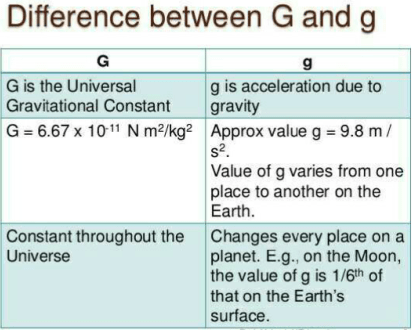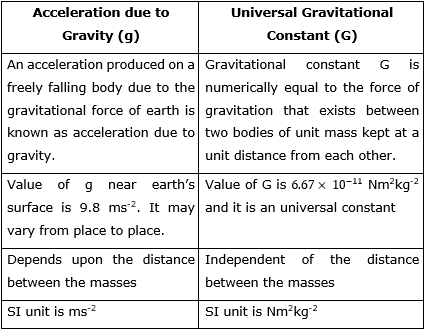Mechanics

# Difference between g and G in tabular formThe basic difference between g and G is that ‘g’ is the Gravitational acceleration while ‘G ‘ is the Gravitational constant. The value of g changes with altitude while the value of G remains constant. Gravitational acceleration is the vector quantity and gravitational constant is the scalar quantity.

## Difference between g and g in Tabular Form### What is the Acceleration of gravity?

The force of gravitational attraction causes an object in free fall on a celestial body to move, dispensing with possible atmospheric resistances, in an accelerated way, that is, with a constant increase in its speed per unit of time, and that it is directed towards the center of the celestial body.

On the surface of the Earth the value of this acceleration, indicated by the letter g, would be the same at any point if our globe were perfectly spherical and if the centrifugal force due to the Earth’s rotation, which has the effect of a decrease in the gravitational force of attraction, had the same value anywhere. When these two conditions are not verified, g varies slightly from place to place.

In Ecuador, the acceleration of gravity is 9.7799 meters per second every second, while at the poles it is greater than 9.83 meters per second every second. The value that is usually accepted internationally for the acceleration of gravity when making calculations is 9.80665 meters per second every second.

Formerly it was believed that the densest bodies fell with greater acceleration, but Galileo and, later, Isaac Newton were in charge of demonstrating the opposite. An experiment conducted in a vacuum chamber demonstrates that all bodies fall toward Earth with the same acceleration, regardless of their mass.

Related topics:
How to calculate the mass of earth?
Difference between mass and weight
If You want to study more related Topics, Then visit Our Page: Mechanics